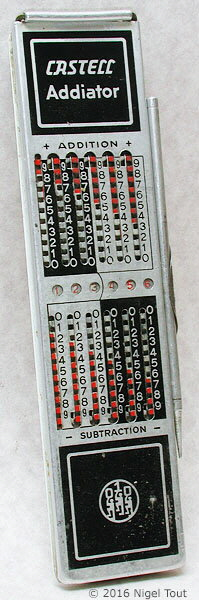Mechanical Calculators

"Addiator" type calculators are very useful for addition and subtraction, whereas slide-rules are very useful for multiplication, division, and more complex functions. By combining the two types in one instrument we obtain a device which can usefully perform the standard 4 functions of addition, subtraction, multiplication, and division.

Known models that are a combined "Addiator" and slide-rule are the Kingson Four-Rule and several models from Faber-Castell, a well known manufacturer of slide rules.

Kingson Four-RuleThe "Addiator" side for addition and subtraction, with the  operating stylus in its holder.The slide-rule side.

Kingson Four-Rule Calculator

Is a Slide-Rule on one side and a 6-decade "Addiator" type calculator on the other side.

For use with totals up to 999,999, or, by putting the decimal point two places from the right, currenccies up to 9,999.99

Addition and subtraction using the "Addiator" type calculator (see the Addiator in this section) and multiplication, division, square roots, and reciprocals using the slide rule.

160 x 40 x 8 mm (6.25" x 1.6" x 0.3").

1960s to early 1970s.

Faber-Castell

Faber-Castell produced several combined Addiator and Slide-rule devices, two of which are shown below.Is a slide-rule on one side and a 6-decade Addiator calculator on the other side.

For use with totals up to 999,999, or, by putting the decimal point two places from the right, currencies up to 9,999.99

Addition and subtraction using the Addiator calculator and multiplication, division, square roots, reciprocals, lgX, x3, x2, and other functions using the slide rule.

158 x 43 x 10 mm (6.25" x 1.6" x 0.3").

1950s to mid 1970s.Faber-Castell 67-87R "RIETZ"

Is a slide-rule on one side and a 6-decade Addiator calculator on the other side.

For use with totals up to 999,999, or, by putting the decimal point two places from the right, currencies up to 9,999.99

Addition and subtraction using the Addiator calculator and multiplication, division, square roots, reciprocals, x2, and other functions using the slide rule.

158 x 42 x 8 mm (6.25" x 1.6" x 0.3").

1950s to mid 1970s.